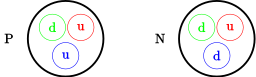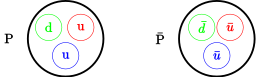# 第一章 粒子介绍

## 1.1 原子、原子核、粒子

1. $\Phi_{Atom} \approx 10^{-10}$ m $\Phi_{Atomic nucleus} \approx 10^{-5} \times \Phi_{Atom}$
2. 质子数 $>$ 电子数，称为离子。从原子中打出一个或多个电子的过程为电离。
3. 上夸克（u） 下夸克 （d）
running...4. 夸克和电子不可观测内部结构，当作点
5. 胶子导致夸克的束缚（质子中子中大部分能量以胶子能量形式存在，电场中能量很小）

## 1.2 光子

1. 一份电磁辐射出的能量
2. 光子能量 无线电$<$红光光子$<$蓝光光子$<$X射线$<\gamma$射线

## 1.3 反粒子

• 电子$\leftrightharpoons$正电子 剧烈反应，湮灭能量
• 特性
1. 每个粒子都存在相应反粒子
2. $m_{Original}=m_{anti}$
3. 其他性质由原粒子相关性质精确确定
• 注意
1. 粒子的反粒子可以为本身（必为纯中性粒子）:例如 光子和反光子
2. 粒子上方加横线代表反粒子：$\overline{Positron}=Electron$ $\overline{Electron}=Positron$
3. 束缚态也有与之相关联的状态
running...## 1.4 质量与能量

1. 质量与引力无关
2. $E = c \sqrt{P^2+m_0^2 c^2}$ 能量，其中P=0时，$E=m_0c^2$
3. $P=\frac{m_0v}{\sqrt{1-\frac{v^2}{c^2}}}$,$E=\frac{m_0c^2}{\sqrt{1-\frac{v^2}{c^2}}}$,$v=\frac{Pc^2}{E}$，其中c=1时 $v=\frac{P}{E}$

## 1.5 事例

1. 一个事例是粒子间发生的一次反应
2. 用某种方法记录下的径迹是事例的记录
3. $n^0\Rightarrow p+e^{-}+\bar{\nu}_{e}$ 反中微子 $\sim$10min 614s
4. 结合能使中子在原子核内不至于衰变
5. 当能量平衡有余量，中子$\beta$衰变
6. 强作用力，短程，比电磁力大.

## 1.6 电子伏与其他单位

1. 1eV=1.602$\times 10^{-19}$J
2. 1eV$\Rightarrow$ 电子经过电势差为1V电场所获得的能量
3. $h=4.13569 \times 10^{-21}$MeV$\cdot s$
4. $E=hv E=mc^2$
5. $\hbar=\frac{h}{2\pi}$ 可选单位使c，$\hbar$为1，自然单位